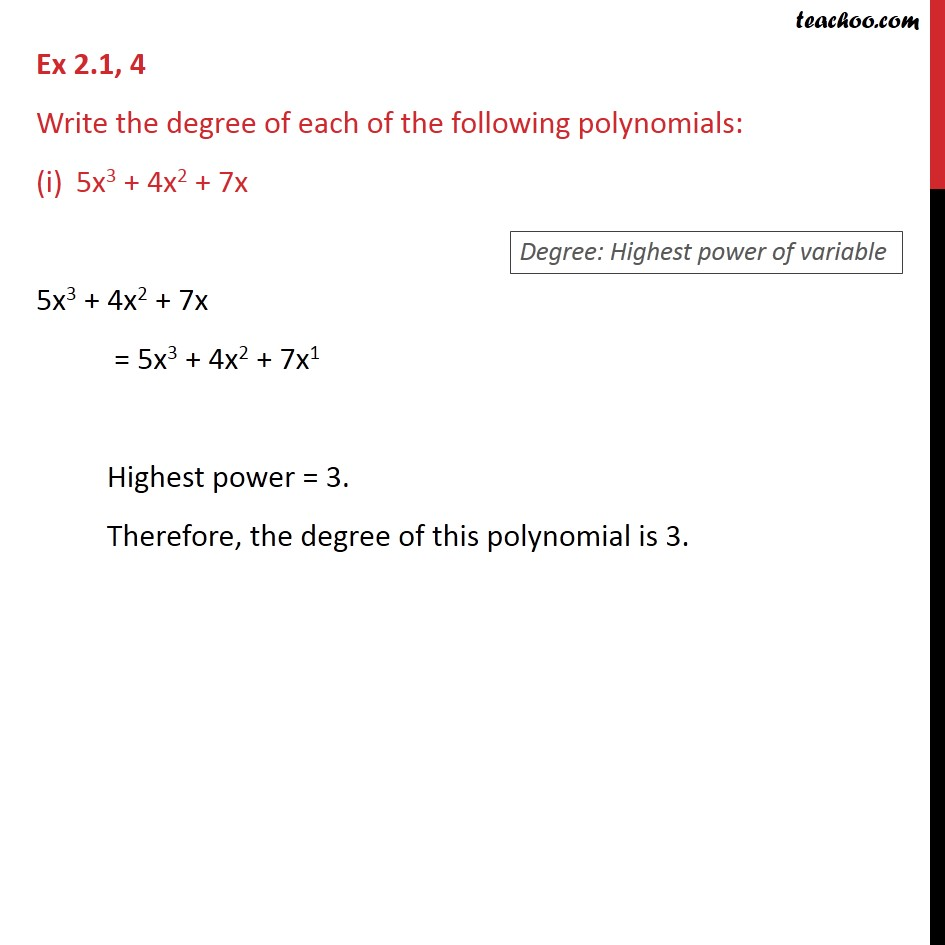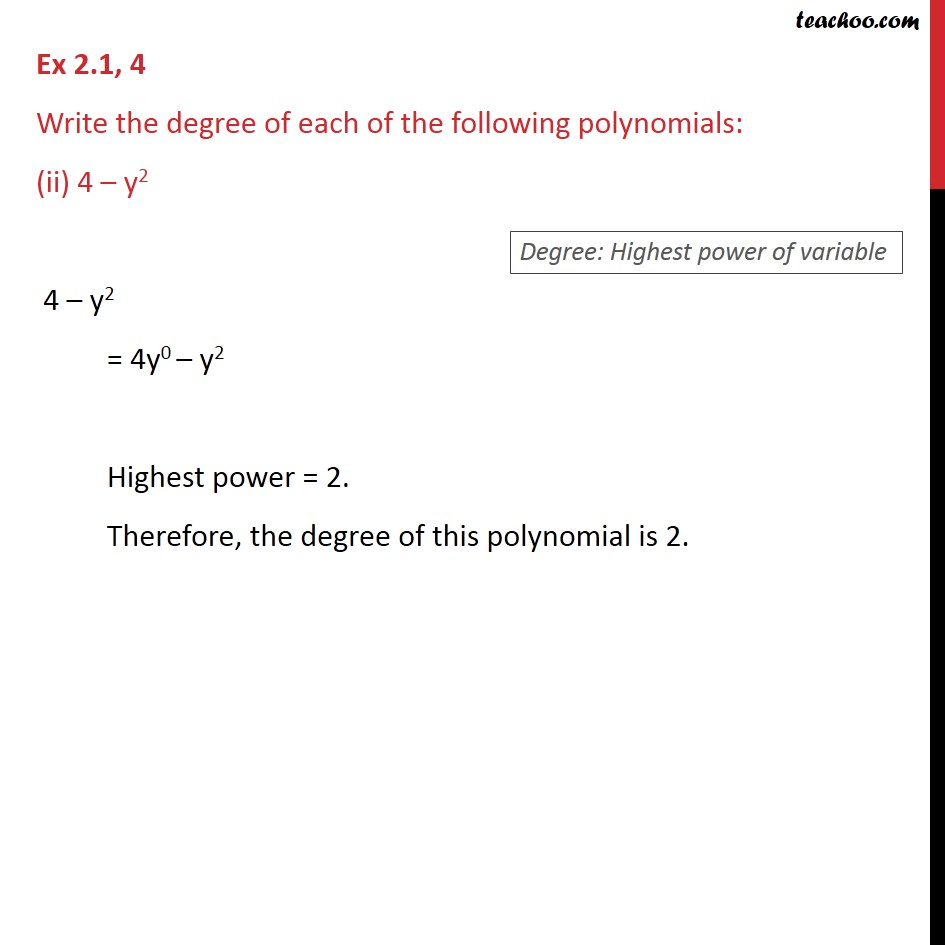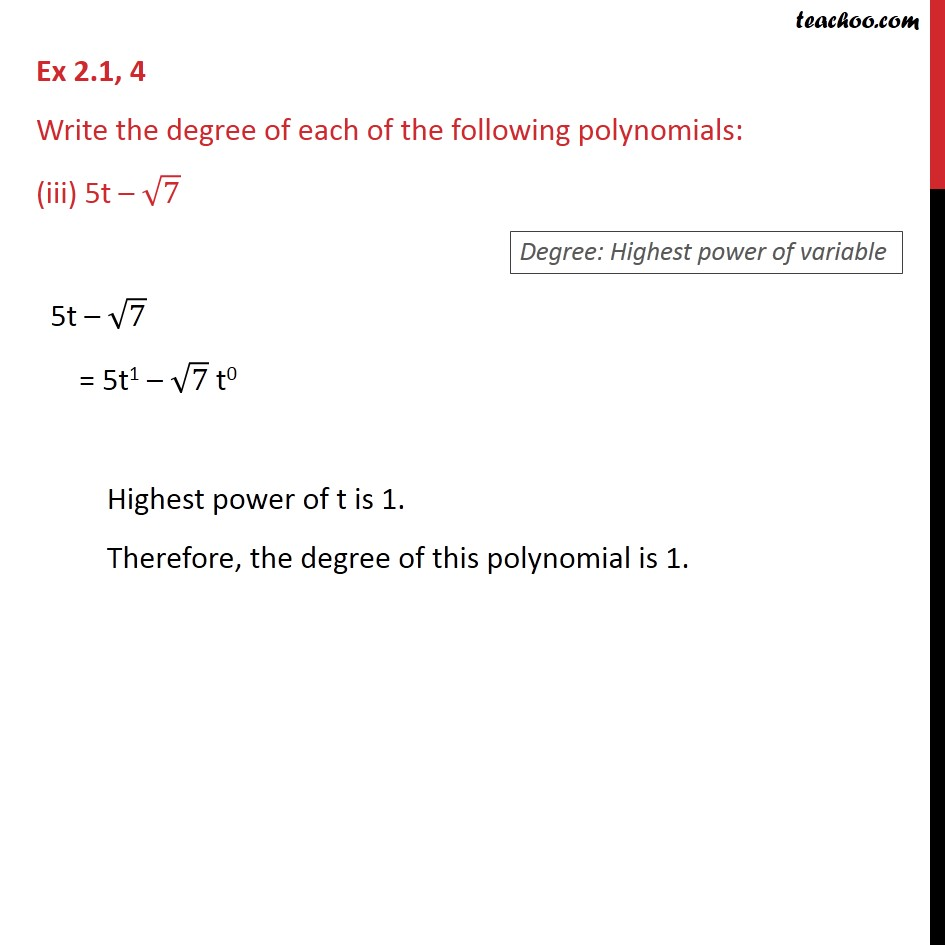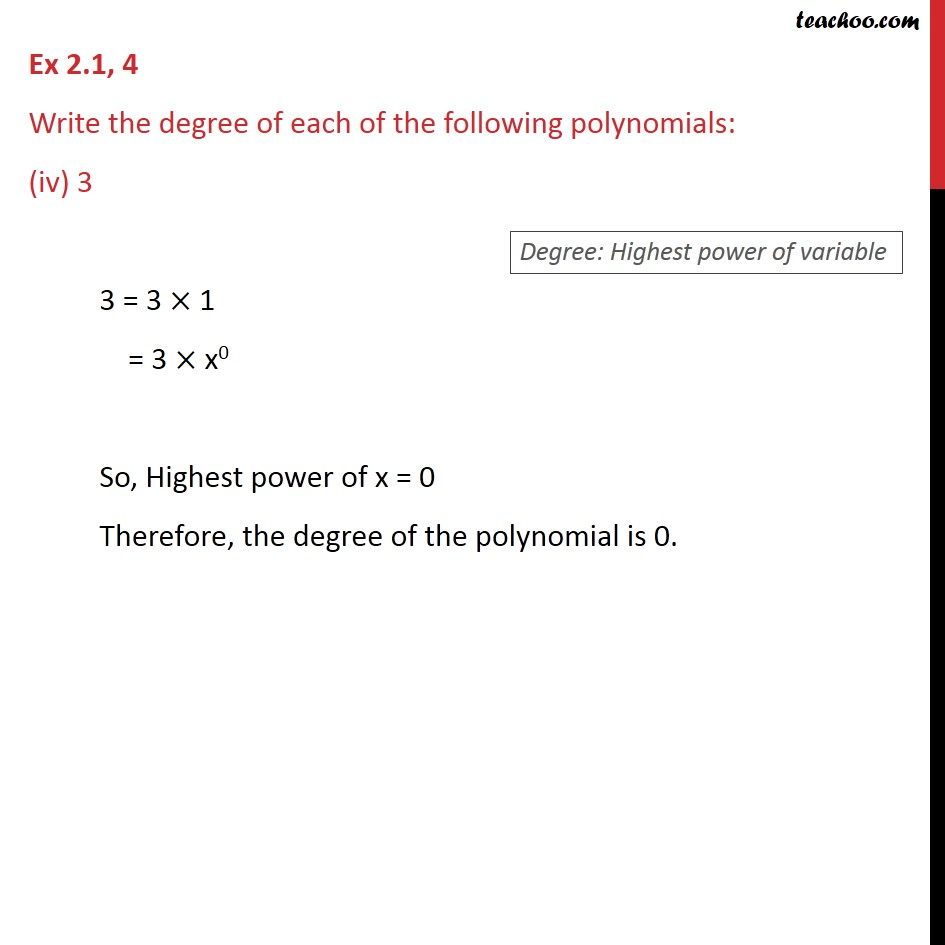Learn all Concepts of Polynomials Class 9 (with VIDEOS). Check - Polynomials Class 91. Chapter 2 Class 9 Polynomials
2. Concept wise
3. Degree & Coefficient of a polynomial

Transcript

Ex 2.1, 4 Write the degree of each of the following polynomials: 5x3 + 4x2 + 7x 5x3 + 4x2 + 7x = 5x3 + 4x2 + 7x1 Highest power = 3. Therefore, the degree of this polynomial is 3. Ex 2.1, 4 Write the degree of each of the following polynomials: (ii) 4 y2 4 y2 = 4y0 y2 Highest power = 2. Therefore, the degree of this polynomial is 2. Ex 2.1, 4 Write the degree of each of the following polynomials: (iii) 5t 7 5t 7 = 5t1 7 t0 Highest power of t is 1. Therefore, the degree of this polynomial is 1. Ex 2.1, 4 Write the degree of each of the following polynomials: (iv) 3 3 = 3 1 = 3 x0 So, Highest power of x = 0 Therefore, the degree of the polynomial is 0.

Degree & Coefficient of a polynomial